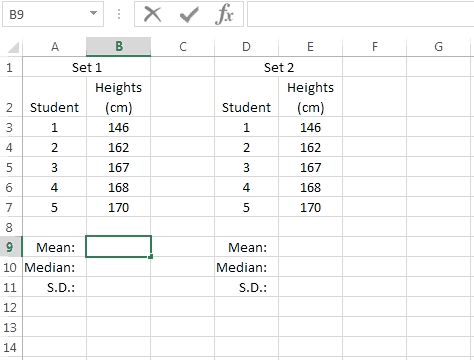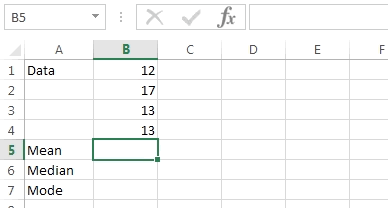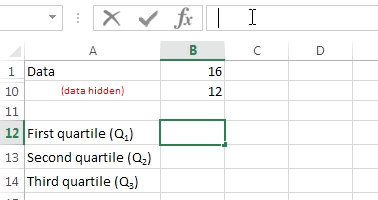### Excel formula

#### Summary Statistics - syllabus example | # gomaths.net/4238

Use a spreadsheet to examine the effect on the calculate summary statistics of changing the value of a score.

Syllabus: Mathematics General Course, page 37

Mean: `=AVERAGE(B3:B7)`

Median: `=MEDIAN(B3:B7)`

S.D.: `=STDEV.P(B3:B7)`

where `B3:B7` is the range of cells containing numerical data.#### Summary Statistics (mean, mode, median) | # gomaths.net/4234

Calculate summary statistics: mean, mode, median

Mean: `=AVERAGE(B1:B4)`

Mode: `=MODE(B1:B4)`

Median: `=MEDIAN(B1:B4)`

where `B1:B4` is the range of cells containing numerical data.#### Summary Statistics (quartiles) | # gomaths.net/4235

Calculate summary statistics: quartiles

First Quartile: `=QUARTILE(B1:B10,1)`

Second Quartile: `=QUARTILE(B1:B10,2)`

Third Quartile: `=QUARTILE(B1:B10,3)`

where `B1:B10` is the range of cells containing numerical data.

Note: Excel calculates quartiles differently to the usual method of splitting the data into 25% lots.